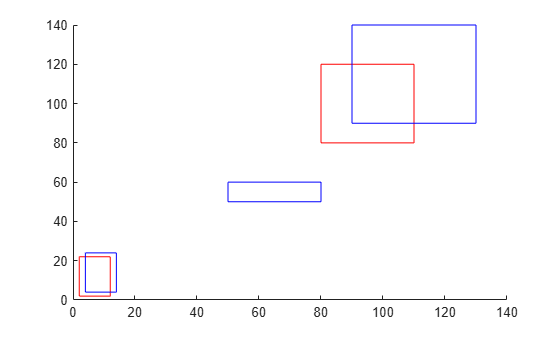# bboxPrecisionRecall

Compute bounding box precision and recall against ground truth

## Syntax

``[precision,recall] = bboxPrecisionRecall(bboxes,groundTruthBboxes)``
``[precision,recall] = bboxPrecisionRecall(bboxes,groundTruthBboxes,threshold)``

## Description

example

````[precision,recall] = bboxPrecisionRecall(bboxes,groundTruthBboxes)` measures the accuracy of bounding box overlap between `bboxes` and `groundTruthBboxes`. Precision is a ratio of true positive instances to all positive instances of objects in the detector, based on the ground truth. Recall is a ratio of true positive instances to the sum of true positives and false negatives in the detector, based on the ground truth.If the bounding box is associated with a class label, `precision` and `recall` contain metrics for each class. If the bounding box is also associated with a confidence score for ranking, use the `evaluateObjectDetection` function.```
````[precision,recall] = bboxPrecisionRecall(bboxes,groundTruthBboxes,threshold)` specifies the overlap threshold for assigning a given box to a ground truth box.```

## Examples

collapse all

Create two ground truth boxes.

`groundTruthBoxes = [2 2 10 20; 80 80 30 40];`

Create three boxes for evaluation.

`boundingBoxes = [4 4 10 20; 50 50 30 10; 90 90 40 50];`

Plot the boxes.

```figure hold on for i=1:2 rectangle('Position',groundTruthBoxes(i,:),'EdgeColor','r'); end for i=1:3 rectangle('Position',boundingBoxes(i,:),'EdgeColor','b'); end ```Evaluate the overlap accuracy against the ground truth data.

`[precision,recall] = bboxPrecisionRecall(boundingBoxes,groundTruthBoxes)`
```precision = 0.3333 ```
```recall = 0.5000 ```

Define class names.

`classNames = ["A","B","C"];`

Create bounding boxes for evaluation.

```predictedLabels = {... categorical("A",classNames); ... categorical(["C";"B"],classNames)}; bboxes = {... [10 10 20 30]; ... [60 18 20 10; 120 120 5 10]}; boundingBoxes = table(bboxes,predictedLabels,'VariableNames',... {'PredictedBoxes','PredictedLabels'});```

Create ground truth boxes.

```A = {[10 10 20 28]; []}; B = {[]; [118 120 5 10]}; C = {[]; [59 19 20 10]}; groundTruthData = table(A,B,C);```

Evaluate overlap accuracy against ground truth data.

`[precision,recall] = bboxPrecisionRecall(boundingBoxes,groundTruthData)`
```precision = 3×1 1 0 1 ```
```recall = 3×1 1 0 1 ```

## Input Arguments

collapse all

Bounding boxes, specified as one of the following. M is the number of bounding boxes.

• For single-class bounding boxes, `bboxes` can be an M-by-4 matrix, or a table with M rows and one column. Each row of the matrix or element in the table represents a bounding box, specified in the format [x y width height], where x and y correspond to the upper left corner of the bounding box.

• For multi-class bounding boxes, `bboxes` is a table with M rows and two columns. Each element in the first column represents a bounding box, specified in the format [x y width height]. The second column contains the predicted label for each box. The label must be a categorical type defined by the variable (column) names of the `groundTruthBboxes` table.

Data Types: `single` | `double` | `int8` | `int16` | `int32` | `uint8` | `uint16` | `uint32`

Ground truth bounding boxes, specified as one of the following. M is the number of ground truth bounding boxes.

• For single-class bounding boxes, `groundTruthBboxes` can be an M-by-4 matrix, or a table with M rows and one column. Each row of the matrix or element in the table represents a bounding box, specified in the format [x y width height], where x and y correspond to the upper left corner of the bounding box.

• For multi-class bounding boxes, `groundTruthBboxes` is a table with M rows and multiple columns. Each column represents a different class, and the column name specifies the class label. Each element in the table has the format [x y width height].

Data Types: `single` | `double` | `int8` | `int16` | `int32` | `uint8` | `uint16` | `uint32`

Overlap threshold for assigned a detection to a ground truth box, specified as a numeric scalar. The overlap ratio is computed as the IoU (intersection over union).

## Output Arguments

collapse all

Precision values from each detection, returned as a numeric scalar for single-class bounding boxes. For multi-class bounding boxes, `precision` is returned as a numeric vector consisting of metrics for each class. The class order follows the same column order as the `groundTruthBboxes` table.

Recall values from each detection, returned as a numeric scalar for single-class bounding boxes. For multi-class bounding boxes, `recall` is returned as a numeric vector consisting of metrics for each class. The class order follows the same column order as the `groundTruthBboxes` table.

## Version History

Introduced in R2018a